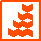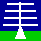HOME

ANNOUNCEMENTS

CONTACTS

DATA

INSTRUMENTS

MISCELLANEOUS

PLOTS

PUBLICATIONS

SCIENCE

WIND-PROFILER
PRINCIPLES

THE NERC MST RADAR FACILITY AT ABERYSTWYTH
FILE FORMAT (BINARY) FOR VERSION-0 MST RADAR TIME-AVERAGED POWER DATAWARNING: Version-0 MST radar data products are DEPRECATED
Users are encouraged to make use of Version-2 Cartesian files.

File contents
These contain radar return signal power data derived from Doppler spectra averaged over 12 minutes. The same data are stored in ASCII format files - please contact the NERC MST Radar Facility Project Scientist for more details.

File naming convention:
pwfYYMMDD.datYYis a 2-digit year [00 - 99] MM is a 2-digit month [01 - 12] DD is a 2-digit day [01 - 31]

Archiving convention: YYYY

File availability
1990 to the present.

File format
The data are recorded in non-standard format binary files as descibed below:

```                        POWER FILES
-----------

File name :- PWFyyMMdd.DAT

RANDOM ACCESS FILE  -  UNFORMATTED
RECORD = 32 BYTES

B = BYTE
I2 = INTEGER*2 (2 byte integer)

DATA IN POWER (PWF) FILE
------------------------

The file contains power data obtained from all the Doppler
spectra files collected on one day.

A Parameter Block for each dwell (beam direction) contains
most of the information from the corresponding parameter block
in the Doppler Spectra file.
It also contains additional information required for file management.

Each Power Data value is the summation of all the values in
the Doppler spectrum obtained for one height.
The spectra are for one dwell (ie one beam direction).
Power Data values require one byte for each height bin and are
expressed as the power to the nearest dB.

The data starts at bin number NH1 and continues to NH2 followed by
NH3 to NH4 if any upper height data was collected in that dwell.
If there is upper height data then NH3 > NH2. Otherwise NH3 = 0.

RECORD ORDERING
---------------

PARAMETER BLOCK             One record containing Dwell Parameters
DATA RECORDS                N records, each with 32 Power values
PARAMETER BLOCK             Parameters for next dwell
DATA RECORDS                Power values for next dwell
etc.

N = 1 + INT(( total number of bins per dwell - 1 ) / 32)

RECORD FORMATS
--------------

Parameter Block ( PB ) ( Start of Each Dwell )
----------------------

Length of Transmitter pulse ( microsec ).          LTX     B
Pulse Code Resolution.                             NCC     B
0 = Uncoded , 1 = 8 uS , 2 = 4 uS
3 = 2 uS , 4 = 1 uS
Pulse Repetion Interval ( microsec ).              IPI     I2
Number of Coherent Pulse Additions.                NPP     I2
Length of FFT.                                     LFT     I2
Number of FFTs Averaged together.                  NAV     B
Beam Number.                                       NBM     B
Receiver Filter (microsec) (1/bandwidth).          NRX     B
Raw Data Collection Flag.                          DMP     B
Negative value if Raw Data File Collected.
YEAR in Form 90,91,92 etc.                         IY      B
MONTH ( 1 - 12 ).                                  IMN     B
DAY ( 1 - 31 ).                                    ID      B
HOUR ( 0 - 23 ).                                   IH      B
MINUTE ( 0 - 59 ).                                 IM      B
SECONDS ( 0 - 59 ).                                IS      B
Start of First Height Range  ( Bin No. ).          NH1     I2
End of First Height Range    ( Bin No. ).          NH2     I2
Start of Second Height Range ( Bin No. ).          NH3     I2
End of Second Height Range   ( Bin No. ).          NH4     I2
Run Number.                                        MST     I2
No. of Cycles * 10 + No. of Dwells in observation. NCD     I2
No. of Records / Cycle.                            NRC     I2

Data Record
-----------

Power value (dB)                                                 B
1 byte / height bin.
32 power values per record.
N records per dwell
Last record of a dwell usually has less than 32 power values.

HEIGHT DERIVATION
-----------------

Height at range bin n is given by :-  Ht = (n - Bz) * HI

Bz is the sea level range bin,
derived as follows from dwell header parameters:
LTX = Transmitter pulse width (microsecs).
If NRX = 1  Bz = 5.7 ( unless LTX = 1 when Bz = 5.2 )
If NRX = 2  Bz = 6.7
If NRX = 4  Bz = 8.7
If NRX = 8  Bz = 12.7

HI is the height increment per bin.
derived as follows from dwell header parameter:
NBM = Antenna beam number.
If NBM = 0               HI = 0.15 Km.    ( Vertical )
If NBM = 1,3,5 or 7      HI = 0.1496 Km.  ( 4.2 Deg. )
If NBM = 2,4,6 or 8      HI = 0.1484 Km.  ( 8.5 Deg. )
If NBM = 9,11,13 or 15   HI = 0.1492 Km.  ( 6.0 Deg. )
If NBM = 10,12,14 or 16  HI = 0.1467 Km.  ( 12.0 Deg.)

Last update of this description 16 February 1995.
```